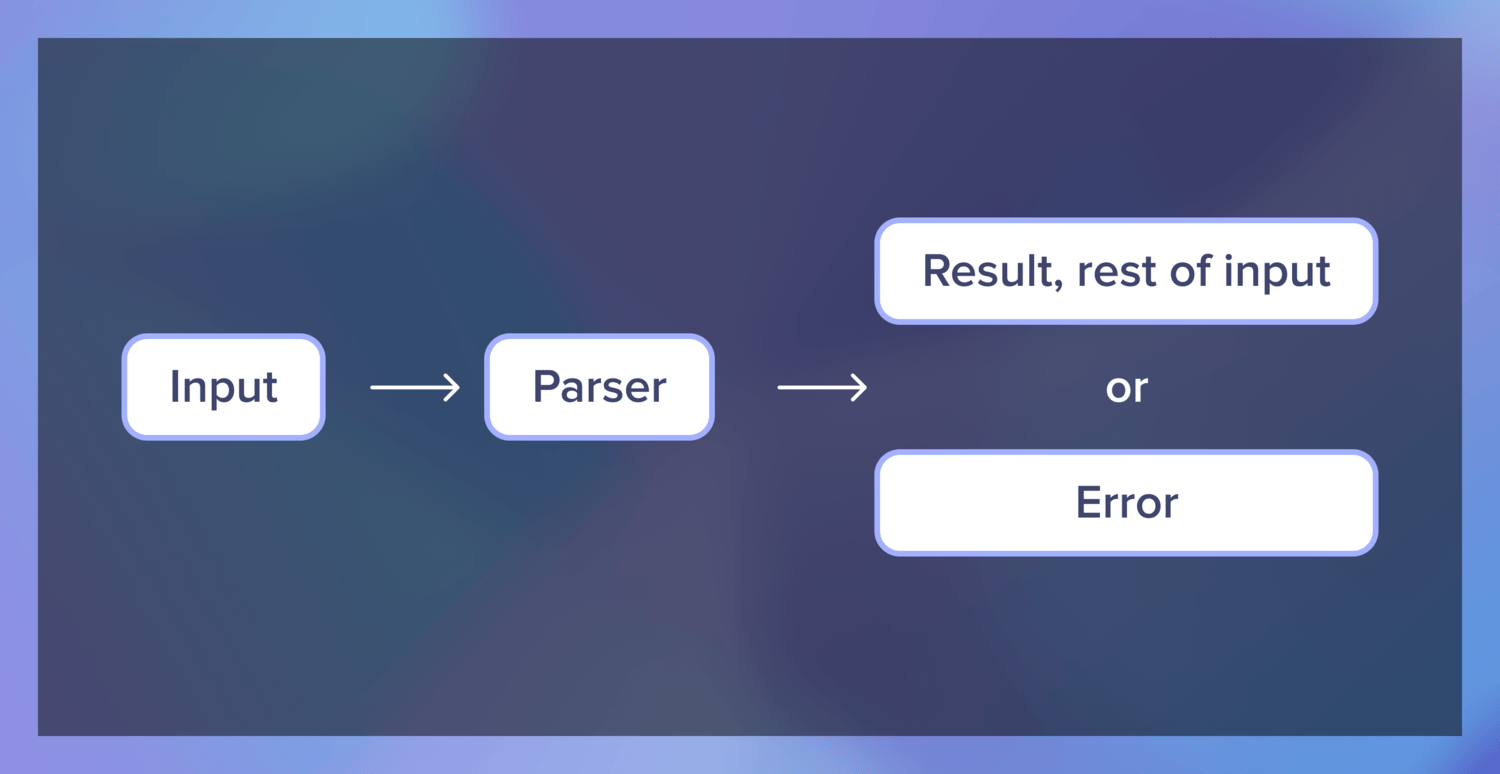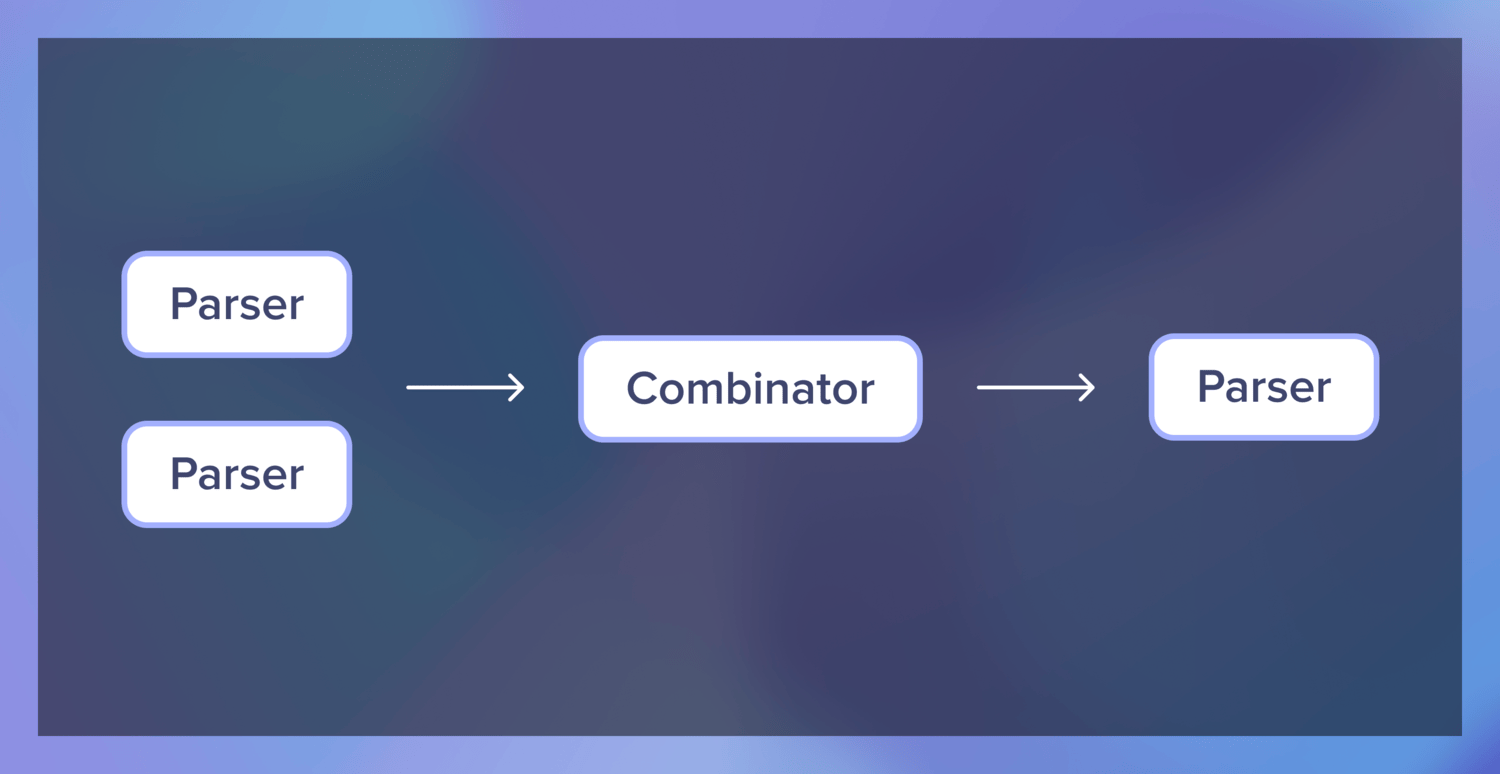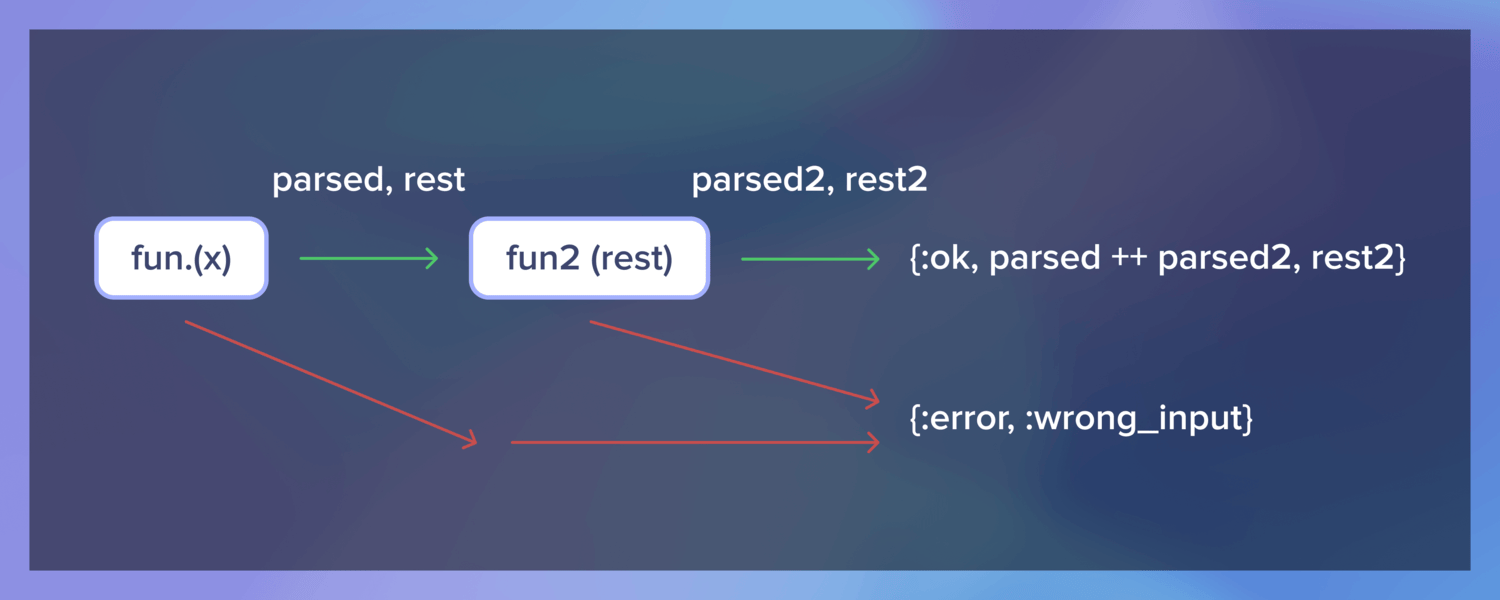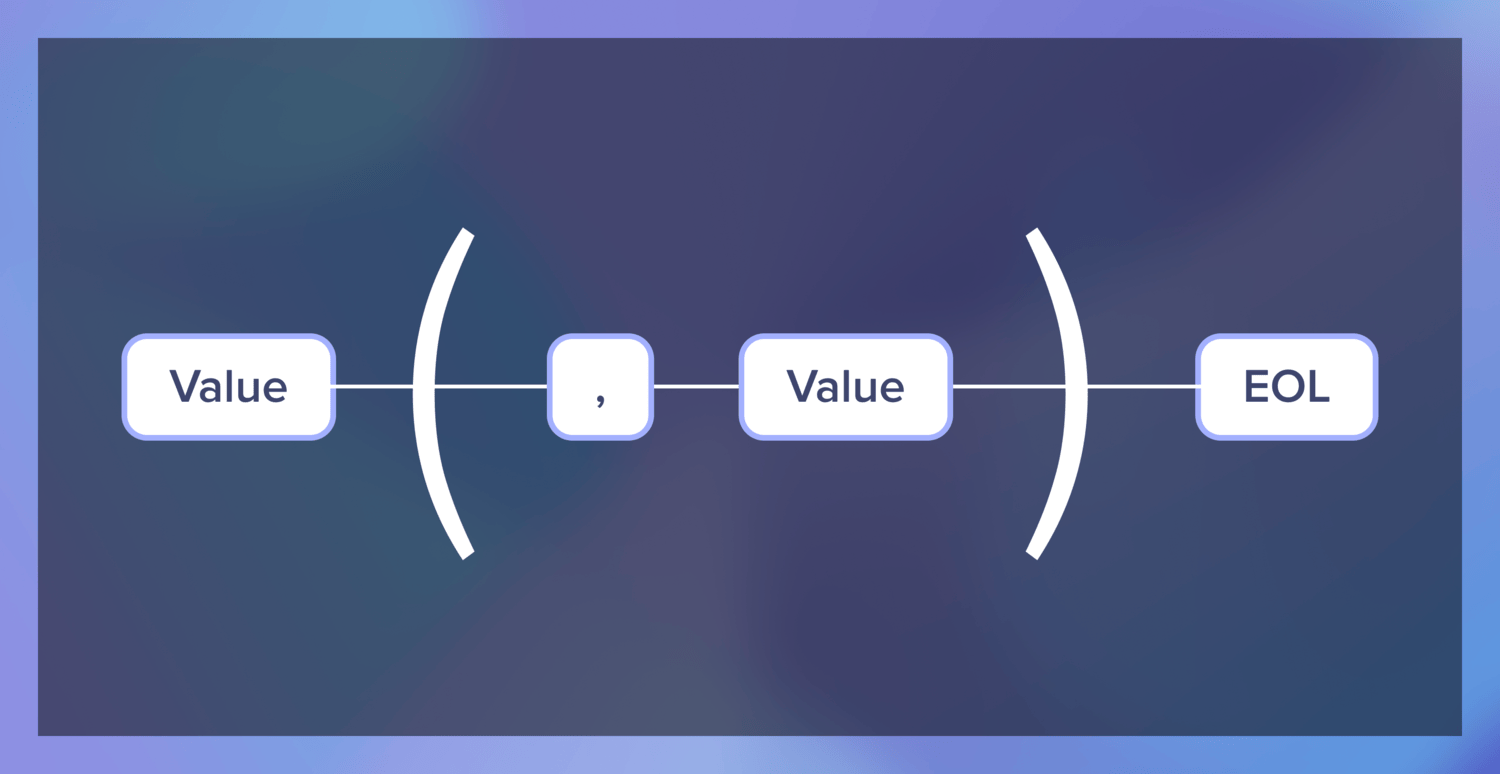# Parser Combinators in Elixir

Parser combinators are one of the most useful tools for parsing. In contrast to regular expressions, they are much more readable and maintainable, making them an excellent choice for more complex tasks.

This article has two parts. First, I’ll explain how parser combinators work and what they are made of. After that, I’ll guide you through making a CSV parser using NimbleParsec, a parser combinator library written in Elixir.

## Introduction to parser combinators

In this part, I’ll give a brief description of parser combinators, and we will try to build functional parser combinators from scratch. The combinators we’ll make will be low-level and worse than what you would get with simple regex; they are there to illustrate the point.

If you want to see parser combinators in action, go straight to the NimbleParsec section.

### What are parser combinators?

When programming, we frequently have to parse input (like a string) into a more computer-friendly data structure (like a tree or a list).

One fast way to do that is to write a regex expression that captures everything we need. But these can become quite lengthy and complicated, which leads to ugly code.

What if we could instead write parsers that map one-to-one to semantical units in the input and combine them to make a parser for that input?

Ultimately, parser combinators are just this: a way to combine simple parsers to create more complex parsers.

### Parsers

So what do parsers do, exactly? The main goal of a parser is to parse a string of text into a different, more structured object, like a list or a tree.

For example, we could accept a list of integers as a string "3, 1, 4, 1" and turn that string into a list to better represent the structure inherent in the string – [3, 1, 4, 1].

But what if we come upon a string like "3, 1, 4, 1 -- Monday, December 28th"? Or "oops, I'm sorry"? To compose with other parsers and handle possible failure, we also need to return the rest of the input if the parser succeeds and an error if it doesn’t.Here’s an example of a low-level parser that parses one decimal digit in Elixir.

  def parse_digit(<<char, rest::bitstring>>) when char >= 48 and char <= 57,
do: {:ok, [char - 48], rest}
def parse_digit(_), do: {:error, :wrong_input}


If you ask what we can do with it, the answer is: not very much. 😅 To unlock the power of parser combinators, we need to find a way to put different parsers together.

### Combinators

A parser combinator is a function that combines two or more parsers into another parser.Let’s think about the ways we could combine parsers. The most straightforward combination would be to link two together – make the parser parse two decimal digits one by one.

  def concat(fun, fun2) do
fn x ->
case fun.(x) do
{:ok, parsed, rest} ->
case fun2.(rest) do
{:ok, parsed2, rest2} ->
{:ok, parsed ++ parsed2, rest2}

err ->
err
end

err ->
err
end
end
end


Here, the resulting parser applies the first function to the input, then the second function to the rest of the input that the first function returns. We return both parsed items as a list and the input that the second function didn’t consume. In case there is an error, it just gets passed further.Now we can use our combinator repeatedly to create a parser that can parse 2, 3, even 4, and more integers in a row! But that’s just the beginning.

There are multiple other combinator possibilities out there. A frequent one is choice, a naive version of which could look like this:

  def choice(fun1, fun2) do
fn x ->
case {fun1.(x), fun2.(x)} do
{{:ok, parsed, rest}, _ } -> {:ok, parsed, rest}
{_, {:ok, parsed, rest}} -> {:ok, parsed, rest}
{err, _} -> err
end
end
end


Here, it would try parsing two different parsers one by one and pick the one that succeeds first or return an error.

Our simple combinators can make a parser that parses either two or three numbers.

  def digit_parser() do
fn x -> parse_digit(x) end
end

def two_digits() do
digit_parser()
|> concat(digit_parser())
end

def three_digits() do
digit_parser()
|> concat(digit_parser())
|> concat(digit_parser())
end

def two_or_three_digits() do
choice(three_digits(), two_digits())
end

iex(1)> SimpleParser.two_or_three_digits.("55")
{:ok, [5, 5], ""}
iex(2)> SimpleParser.two_or_three_digits.("5a")
{:error, :wrong_input}


By combining different parsers, you can build large, complicated parsers that represent the rules of languages like JSON or XML, for example.

Real parser combinator libraries usually offer an assortment of different combinators that make it possible to represent parsers in a readable manner. We’ll see that later in our NimbleParser example.

### Error handling in parser combinators

Our preliminary error handling is rather naive, and I’ve been informed that there is a misconception that parser combinators handle errors badly. Let’s see how we can easily extend our parser to show the position of unexpected input.

First, let’s change how parse_digit handles errors:

  def parse_digit(<<char, rest::bitstring>>) when char >= 48 and char <= 57,
do: {:ok, [char - 48], rest}

def parse_digit(<<char, _rest::bitstring>>), do: {:error, {:unexpected_input, <<char>>, 1}}
def parse_digit(""), do: {:error, :end_of_string}
def parse_digit(_), do: {:error, :not_string}


In addition to input errors, an EOS error can happen quite easily, so I made sure to cover that.

Now we can modify our concat combinator to track the position of an input error, if it occurs:

  def concat(fun, fun2) do
fn x ->
case fun.(x) do
{:ok, parsed, rest} ->
case fun2.(rest) do
{:ok, parsed2, rest2} ->
{:ok, parsed ++ parsed2, rest2}

{:error, {:unexpected_input, input, pos}} ->
{:error, {:unexpected_input, input, String.length(x) - String.length(rest) + pos}}

err ->
err
end

err ->
err
end
end
end


The choice combinator already handles these errors well. You can see the end result here.

Now when we try to do two_or_three_digits.("5a"), we’ll get {:error, {:unexpected_input, "a", 2}}. If we expose the code as a library, we can easily make nice error messages.

This code is, of course, for demonstration purposes only, but a similar approach is used in megaparsec, a Haskell parser combinator library that is renowned for its decent error reporting.

### Where can you use parser combinators?

Since parser combinators are much more powerful than regex, you can use them for parsing items with complex, recursive structures. But they can also be used for simple parsers where an item can have a lot of different alternatives, for example.

They do not replace regex, though. Each tool has its benefits. I would use regex for simple scripts or one-liners and parser combinators for most other parsing needs.

Quick aside: I got helped a lot in this section by Jonn Mostovoy, who recently published a hands-on guide to using parser combinators in Rust. If you’re interested in seeing how to handle them in a bare metal language, I’d suggest checking it out.

## Create your own CSV parser with NimbleParsec

NimbleParsec is a library that uses metaprogramming to provide you with efficient parsers that compile to binary pattern matching. In this section, we’ll use it to build a simple CSV parser that will take a CSV file and convert it into a list of lists.

### Setup

First off, let’s use mix new CSVParser to create a new project called CSVParser. Afterward, add {:nimble_parsec, "~> 1.0"} to the list of dependencies in mix.exs and import NimbleParsec in the module.

defmodule CSVParser do
import NimbleParsec

end


### Grammar

Now we have to think about the structure of a CSV file.

CSV consists of lines, each of which consists of values separated by commas. We can probably define a CSV value and then use the definition to define a line. Let’s write out simple definitions in English.

• Value is a string (let’s ignore numbers, escape characters, and floats for now).
• Line consists of a value, then possible repeats of (comma, then value), then an EOL character.

How can we use the functions available in the library to reflect this simple grammar?

### Building blocks

Let’s try to build individual parsers for value and line.

To implement value, we need to think about the characters that will separate these values. One good contender would be ,, but you can also encounter newline characters \n and \r. Values can also be empty, so we need to provide for that.

The best fit for our goal is utf8_string, which lets us provide several arguments such as not (which chars not to parse) and min (minimum length).

value = utf8_string([not: ?\r, not: ?\n, not: ?,], min: 0)


Then, we need to define a line. For us, a line is a value, then a comma and a value, repeated 0 or more times, and then an EOL character.We have the value defined, but let’s quickly define an EOL parser that covers Windows, macOS, and Linux.

  eol =
choice([
string("\r\n"),
string("\n")
])


As we saw before, choice enables us to parse the first option that succeeds from a list of functions.

After that, we can use the combinators ignore, concat, and repeat together with our defined parsers to define a line.

  line =
value
|> repeat(ignore(string(",")) |> concat(value))
|> ignore(eol)


ignore will ignore the character and move forward without parsing anything, concat composes two parsers, and repeat repeats a parser until it doesn’t succeed.

### Putting it together

Now that we have the line element, it is very easy to define the full parser. To do that, we need to use the defparsec macro.

defparsec :file, line |> wrap() |> repeat(), debug: true


Here, we parse a line, wrap it in [], and repeat the process until it doesn’t succeed. Now, if we read a CSV file, CSVParser.file(file_contents) will parse the contents of simple CSV files.

Here’s all the code:

defmodule CSVParser do
import NimbleParsec

value = utf8_string([not: ?\r, not: ?\n, not: ?,], min: 0)

eol =
choice([
string("\r\n"),
string("\n")
])

line =
value
|> repeat(ignore(string(",")) |> concat(value))
|> ignore(eol)

defparsec :file, line |> wrap() |> repeat(), debug: true
end


Hooray, a working CSV parser!

Or is it? 🤔

### Preparing for escape

Our CSV definition was very simple. It didn’t cover some of the things that can appear in CSV, and it also treats numbers and strings the same. Arguably, we could have split the file on newline chars, mapped a split on commas on the resulting list, and achieved the same result.

But, since we have created a good foundation, adding new definitions to the parser is much simpler than improving a two-line function. Let’s try to fix one of these issues now.

One of the problems is that CSV files can have commas in entries. Our parser always splits on the comma. Let’s add an option to escape commas by wrapping the entry in double quotes.

To do that, we need to extend our value definition.

An escaped value consists of zero or more characters, surrounded by double quotes. In case there is a double quote inside the escaped value, the double quote will need to be escaped by another double quote.

In other words, these are all valid options:

text
"text"
"text, text"
"text, ""text"""


First of all, our item is surrounded by double quotes.

  escaped_value =
ignore(string("\""))
???
ignore(string("\""))


Then we need to figure out how to parse the inside of the double quotes to fulfill the requirements.

After going through a rather roundabout way to achieve this (you don’t want to know 🙈), I found a tip in Real World Haskell that we can just read characters of the item one by one, matching only two double quotes in a row or a non-quote character.

This would parse one character:

  escaped_character =
choice([
string("\"\""),
utf8_string([not: ?"], 1)
])


Now we can use the repeat combinator on escaped_character, then join all the characters we parsed.

  escaped_value =
ignore(string("\""))
|> repeat(escaped_character)
|> ignore(string("\""))
|> reduce({Enum, :join, [""]})


Let’s rename the original value to regular_value and make value a choice between escaped_value and regular_value.

  escaped_character =
choice([
string("\"\""),
utf8_string([not: ?"], 1)
])

regular_value = utf8_string([not: ?\r, not: ?\n, not: ?,], min: 0)

escaped_value =
ignore(string("\""))
|> repeat(escaped_character)
|> ignore(string("\""))
|> reduce({Enum, :join, [""]})

value =
choice([
escaped_value,
regular_value
])


We need to put escaped_value first because otherwise, the parser will succeed with regular_value on our string before we get a chance to escape.

You can see the full parser code here.

### Further improvements

This parser can be further improved, of course. For example, you can add support for extra whitespace or numbers, which is an exciting exercise to do on your own.

I hope that this has been an exciting journey and that you learned something new today! If you want to read more about Elixir, you are welcome to browse our Elixir articles and follow us on Twitter, DEV or Medium to receive updates whenever we publish new ones.